### IMO Shortlist 2006 problem C1

Kvaliteta:
Avg: 4,0
Težina:
Avg: 6,0
We have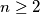$n \geq 2$ lamps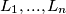$L_{1}, . . . ,L_{n}$ in a row, each of them being either on or off. Every second we simultaneously modify the state of each lamp as follows: if the lamp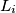$L_{i}$ and its neighbours (only one neighbour for$i = 1$ or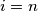$i = n$, two neighbours for other$i$) are in the same state, then$L_{i}$ is switched off; – otherwise,$L_{i}$ is switched on.
Initially all the lamps are off except the leftmost one which is on.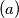$(a)$ Prove that there are infinitely many integers$n$ for which all the lamps will eventually be off.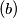$(b)$ Prove that there are infinitely many integers$n$ for which the lamps will never be all off.
Izvor: Međunarodna matematička olimpijada, shortlist 2006